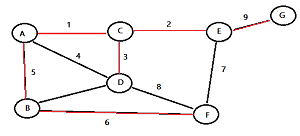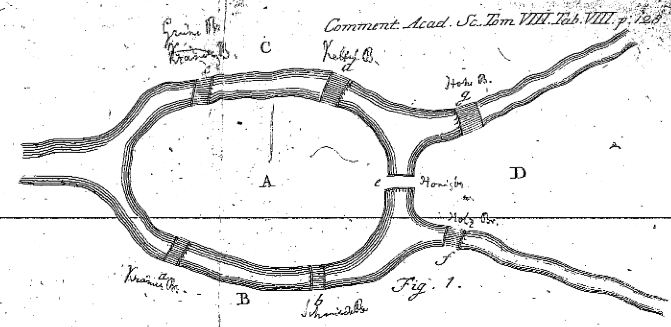# Graph Theory: Definitions for Common Terms

Share on

Graph Theory is the study of lines and points.

It is a sub-field of mathematics which deals with graphs: diagrams that involve points and lines and which often pictorially represent mathematical truths. Graph theory is the study of the relationship between edges and vertices. Formally, a graph is a pair (V, E), where V is a finite set of vertices and E a finite set of edges.A minimum spanning tree. The edges form straight lines between vertices (nodes).

## Definitions in Graph Theory

Graph Theory has some unique vocabulary:

• An arc is a directed line (a pair of ordered vertices).
• An edge is line joining a pair of nodes.
• Incident edges are edges which share a vertex. A edge and vertex are incident if the edge connects the vertex to another.
• A loop is an edge or arc that joins a vertex to itself.
• A vertex, sometimes called a node, is a point or circle. It is the fundamental unit from which graphs are made.
• Adjacent vertices are vertices which are connected by an edge.
• The degree of a vertex is simply the number of edges that connect to that vertex. Loops count twice.
• A predecessor is the node (vertex) before a given vertex on a path.
• A successor is the node (vertex) following a given vertex on a path.
• A walk is a series of vertices and edges.
• A circuit is a closed walk with every edge distinct.
• A closed walk is a walk from a vertex back to itself; a series of vertices and edges which begins and ends at the same place.
• A cycle is a closed walk with no repeated vertices (except that the first and last vertices are the same).
• A path is a walk where no repeated vertices. A u-v path is a path beginning at u and ending at v.
• A u-v walk would be a walk beginning at u and ending at v.

## More Definitions:

• An edge contraction involves removing an edge from a graph by merging the two vertices it used to join.
• In computer science and computer-based graph theory, a graph traversal is an exploration of a graph in which the vertices are visited or updated one by one.
• A Hamiltonian cycle is a closed loop where every node is visited exactly once.

## Types of Graphs

• An acyclic directed graph is a finite directed graph which has no directed cycles.
• A directed graph is a graph where the edges have direction; that is, they are ordered pairs of vertices.
• A condensation of a multigraph is the graph that results when you delete any multiple edges, leaving just one edge between any two points.
• If a graph has a path between every pair of vertices (there is no vertex not connected with an edge), the graph is called a connected graph.
• If a graph G’ can be constructed from a graph G by repeated edge contractions or deletions, the graph G’ is a graph minor of G.
• An inverted graph G’ of G is a graph with the same vertices but none of the same edges; two vertices in G’ are adjacent if and only if they were not adjacent in G.
• A multigraph is a graph without loops, but which may have multiple edges.
• A null graph is a graph with no edges. It may have one or more vertices.
• An oriented graph is a directed graph that doesn’t have any symmetric pairs of directed edges.
• A simple graph is a graph that doesn’t have any loops or multiple edges. No multiple edges means that no two edges have the same endpoints.
• A subgraph is a graph whose vertices and edges are included in the vertices and edges of another graph (the supergraph).
• A symmetric graph is a directed graph D where, for every arc (x,y), the inverted arc (y,x) is also in D.
• A trivial graph is a graph with only one vertex.
• An undirected graph is a graph where none of the edges have direction; the pairs of vertices that make up each edge are unordered.

## Graph Theory in History

Graph Theory dates back to 1735 and Euler’s Seven Bridges of Königsberg. The city of Königsberg was a town with two islands, connected to each other and to the mainland by seven bridges. The question set was whether it were possible to take a walk and cross each bridge exactly once. In a first demonstration of graph theory, Euler showed that it was not possible.From: ‘Solutio problematis ad geometriam situs pertinentis,’ Eneström 53 [source: MAA Euler Archive]

## Mantel’s Theorem

Mantel’s theorem, published in 1907, tells us the largest number of edges a graph with a given number of vertices may have without having a triangle for a subgraph. It can be stated as:

A graph with n vertices and m edges will contain a triangle as a subgraph if and only if m > n2/4.

## References

Introduction to Combinatorics and Graph Theory. Retrieved 8/11/2017 from: https://www.whitman.edu/mathematics/cgt_online/cgt.pdf
Turan’s Theorem
Mathematics of Bioinformatics

CITE THIS AS:
Stephanie Glen. "Graph Theory: Definitions for Common Terms" From StatisticsHowTo.com: Elementary Statistics for the rest of us! https://www.statisticshowto.com/graph-theory/
---------------------------------------------------------------------------Need help with a homework or test question? With Chegg Study, you can get step-by-step solutions to your questions from an expert in the field. Your first 30 minutes with a Chegg tutor is free!